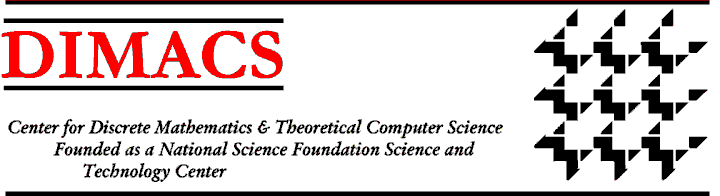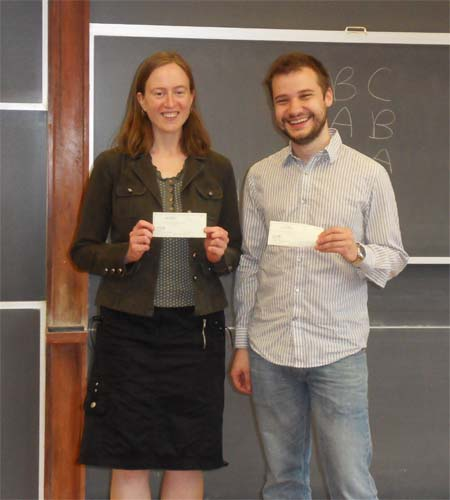The Discrepancy of Three Permutations[October, 2012] DIMACS researcher Alantha Newman and graduate student Aleksandar Nikolov discovered a counterexample to a long-standing a conjecture by Jozsef Beck (Rutgers) on the discrepancy of a set system arising from three permutations on the integers 1 through n. Given a set system (i.e., m sets on n elements where m is O(n)), the problem is to assign each element a value of 1 or -1 so as to minimize the maximum over all sets of the absolute value of the sum of the values assigned to its elements. This minimum is the discrepancy.

Beck’s “Three Permutations Conjecture” (c. 1982) states that when the set system is derived from three permutations, the discrepancy of the set system is a constant. The analogous result is true for the set system based on two permutations, and there is a simple, well-known proof demonstrating that fact.

Newman and Nikolov showed that this well studied conjecture is false for three permutations by providing a counterexample.  For any positive integer n =3k , they exhibited three permutations whose corresponding set system has discrepancy(log(n)).

Professor Joel Spencer (NYU) stated Beck’s conjecture in his Ten Lectures on the Probabilistic Method in 1987 and offered a \$100 reward for settling it. Newman and Nikolov are shown collecting this bounty.

One inspiration for Newman and Nikolov’s work was a paper by Eisenbrand, Palvolgyi and Rothvoss (SODA 2010) that showed that if Beck’s conjecture were true, then a well-studied linear programming (LP) relaxation for bin packing would yield a lower bound on the value of an optimal integer solution that is within an additive constant of optimal. Newman and Nikolov’s result closes that particular path to approximability of bin packing.

Subsequently, in joint work with Ofer Neiman (then a postdoctoral researcher at Princeton), Newman and Nikolov showed that this counterexample can nevertheless be used to construct bin packing instances with interesting consequences. These instances have corresponding optimal LP solutions that can be used (under a particular natural restriction that holds, for example, in the Karmarker-Karp algorithm for bin packing) to obtain integral solutions that use(log(n)) more bins than that of an optimal solution. Eisenbrand et al. independently made this observation in an extended version of their original paper, while Newman, Neiman and Nikolov presented their work at the 2012 FOCS conference in New Brunswick.